## Accelerate Simulation Speed by Using GPU Coder

With GPU Coder™, you can speed up the execution of Simulink® models that contain MATLAB Function (Simulink) blocks. GPU-accelerated simulation also works with models that contain blocks from the Deep Neural Networks library of the Deep Learning Toolbox™ or the Analysis and Enhancement library from the Computer Vision Toolbox™. GPU-accelerated simulation requires a CUDA®-enabled NVIDIA® GPU.

When you enable GPU-accelerated simulation, the software partitions and generates CUDA MATLAB® executable (MEX) code from the MATLAB Function blocks and dynamically links the generated code to Simulink during simulation. The generated code contains CUDA kernels that runs on thousands of GPU cores in parallel. It also contains sequential sections of code that run on the CPU.

To perform GPU-accelerated simulation, you:

• Create or open a model. Move the computationally intensive portions of your application into MATLAB Function blocks

• Select the Solver, Language, and other GPU-specific configuration parameters.

• Run the GPU-accelerated model.

### Accelerate Sobel Edge Detection

This example shows how to perform GPU-accelerated simulation of a Sobel edge detection model. The edge detection model uses MATLAB Function blocks to implement the detection algorithm.

Sobel Edge Detection

The Sobel edge detection algorithm performs a 2-D spatial gradient operation on a grayscale image. This algorithm emphasizes the high spatial frequency regions that correspond to the edges of the input image.

The Sobel edge algorithm computes the horizontal gradient, `H` and the vertical gradient, `V` of the input image by using two orthogonal filter kernels, `k` and `k'`. After the filtering operation, the algorithm computes the gradient magnitude and applies a threshold to find the regions of the images identified as edges.

```img = imread('peppers.png'); threshold = 100; k = single([1 2 1; 0 0 0; -1 -2 -1]); H = conv2(img(:,:,2),k, 'same'); V = conv2(img(:,:,2),k','same'); E = sqrt(H.*H + V.*V); edgeImage = uint8((E > threshold) * 255); h = figure; h.Position(3) = 2*h.Position(3); ax1 = subplot(1,2,1); ax2 = subplot(1,2,2); image(ax1,img); xticks(ax1,[]); yticks(ax1,[]) title(ax1,'Test Image') image(ax2,repmat(edgeImage,[1 1 3])); xticks(ax2,[]); yticks(ax2,[]) title(ax2,'Edge Detected Image') ```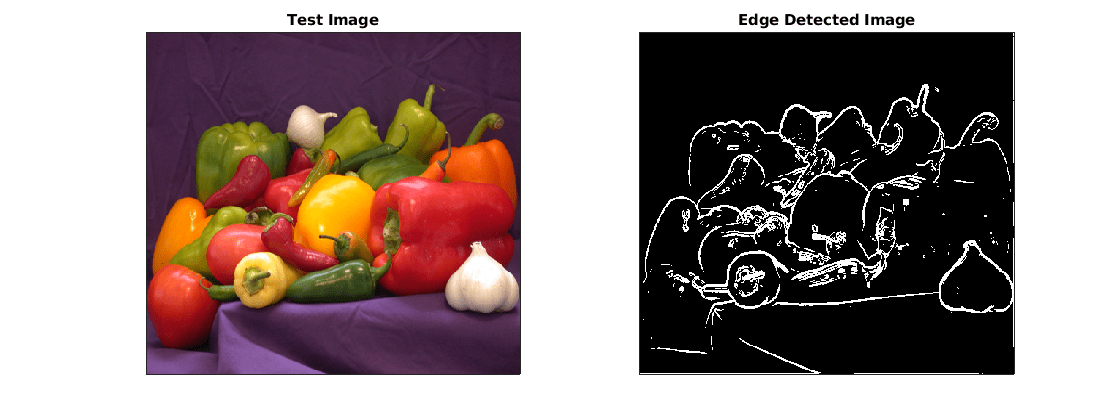Create Edge Detection Model

1. Create a Simulink model and insert two MATLAB Function blocks from the User-Defined Functions library.

2. Add a Constant block and set its value to `0.4`.

3. Add a From Multimedia File block from the Computer Vision Toolbox™ library.

4. Open the From Multimedia File block and set the File name parameter to `rhinos.avi`. Set the Image signal parameter to `One multidimensional signal`.

5. Add two Video Viewer blocks from the Computer Vision Toolbox library to the model.

To open a model that contains these blocks, enter:

```open_system("edgeDetectionInitial") ```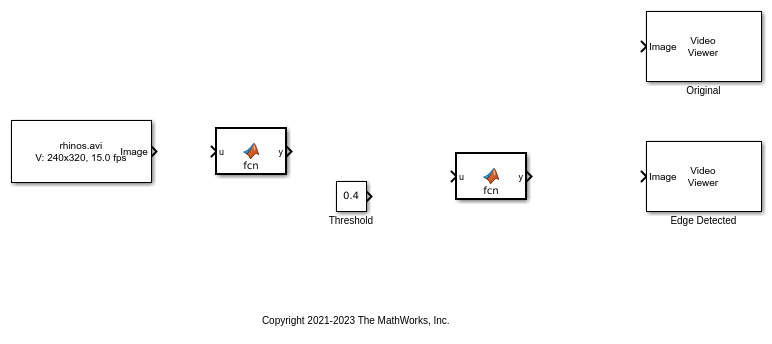6. Double-click one of the MATLAB Function blocks. A default function signature appears in the MATLAB Function Block Editor.

7. Define a function called `sobel` that implements the Sobel edge detection algorithm. The function header declares `grayImage` and `threshold` as input arguments to the `sobel` function and `edgeImage` as the return value.

Save Editor document.

```function edgeImage = sobel(grayImage,threshold) %#codegen ```
```% Define Kernel for Sobel edge detection k = single([1 2 1; 0 0 0; -1 -2 -1]); ```
```% Detect Edge H = conv2(single(grayImage),k, 'same'); V = conv2(single(grayImage),k','same'); E = sqrt(H.*H + V.*V); ```
```edgeImage = uint8((E > threshold) * 255); ```
```end ```

8. Open the block parameters for the MATLAB Function block. On the Code Generation tab, set the Function packaging parameter to `Reusable function`. If the Function packaging parameter is set to any other value, CUDA kernels may not get generated.

9. Modify the other MATLAB Function block to implement the RGB-to-grayscale conversion. Set the Function packaging parameter of the MATLAB Function block to `Reusable function`.

```function gray = RGB2gray(RGB) %#codegen ```
```% Convert color image to grey image gray = (0.2989 * double(RGB(:,:,1)) + ... 0.5870 * double(RGB(:,:,2)) + ... 0.1140 * double(RGB(:,:,3))); ```
```end ```

10. Connect these blocks as shown in the diagram. Save the model as `edgeDetection.slx`. To open a preconfigured model, enter:

```open_system("edgeDetection"); ```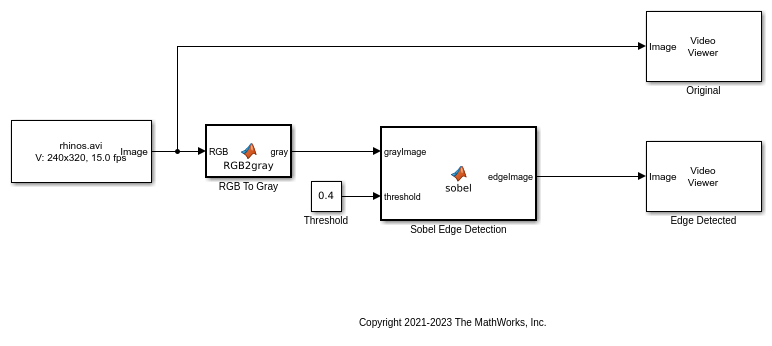11. To test the model for errors, simulate the model. On the toolstrip, click Run.

To see all video frames during simulation, open the Video Viewer block and disable Simulation > Drop Frames to Improve Performance.

```set_param('edgeDetection', 'SimulationMode', 'Normal'); sim('edgeDetection'); ```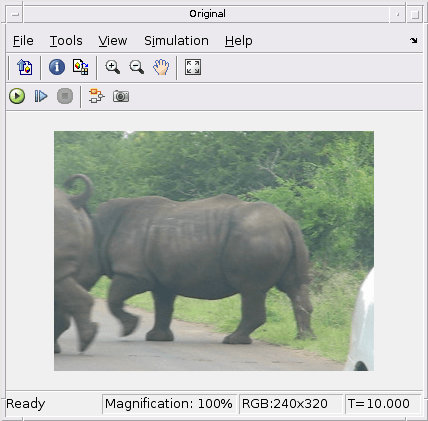Configure Model for GPU Acceleration

The Model configuration parameters determine the acceleration method used during simulation.

1. Open the Configuration Parameters dialog box. Open the Solver pane. To compile your model for acceleration and generate CUDA code, configure the model to use a fixed-step solver. This table shows the solver configuration for this example.

2. In the left-pane, click Simulation Target then enable GPU acceleration parameter.

Note: The Language parameter is automatically set to C++.

3. Optionally, click Simulation Target > GPU Acceleration to view and modify GPU-specific options. For the purposes of this example, you can use the default values for all these parameters.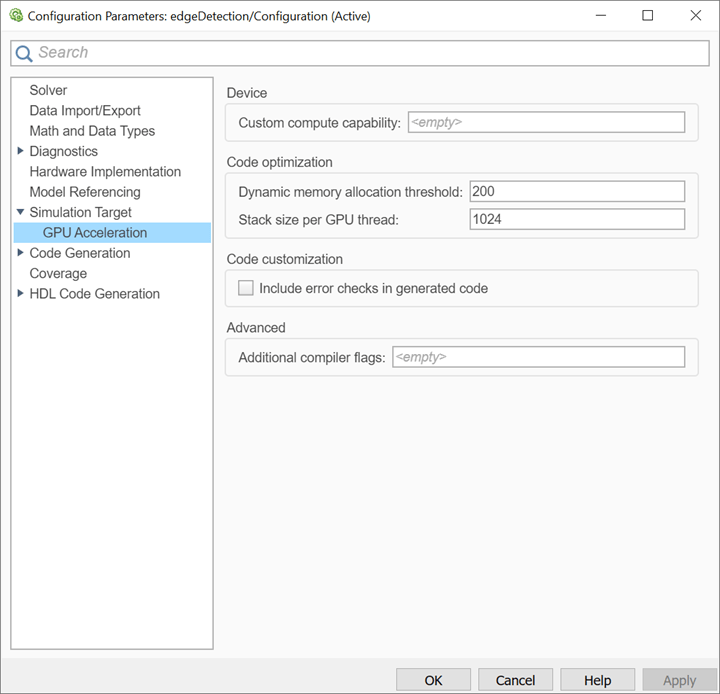4. To save and close the Configuration Parameters dialog box, click OK.

Alternatively, use the `set_param` function to configure the model parameters programmatically in the MATLAB command Window.

```set_param('edgeDetection','SolverType','Fixed-step'); set_param('edgeDetection','SolverName','FixedStepDiscrete'); set_param('edgeDetection','FixedStep','auto'); set_param('edgeDetection','GPUAcceleration','on'); ```

Build GPU-Accelerated Model

To build and simulate the GPU-accelerated model, click Run or use this command:

```sim('edgeDetection'); ```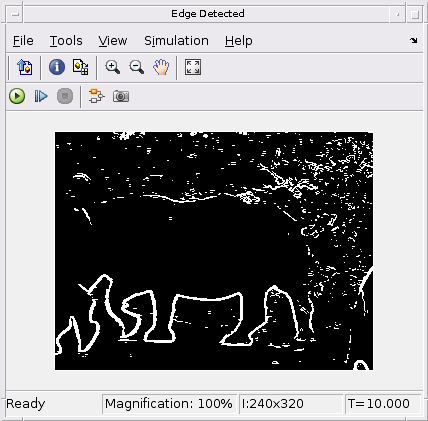The software first checks to see if CUDA code was previously compiled for the model. If code was created previously, the software runs the model. If code was not previously built, the software first generates and compiles the CUDA code, and then runs the model. The code generation tool places the generated code in a subfolder of the working folder called `slprj/_slprj/edgeDetection`.

### Limitations

• GPU code generation for MATLAB Function in Stateflow® charts is not supported.

• When you enable GPU acceleration, you cannot use the Simulation Target > Advanced parameters > Import custom code parameter to import custom CUDA source files (*.cu). Instead, use `coder.ceval` inside the MATLAB Function block.

• The MATLAB Function block does not support all the data types from the MATLAB language. For supported data types, see MATLAB Function (Simulink).